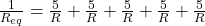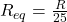Question

A metal wire of resistance R is cut into five equal pieces that are then placed together side by side to form a new cable with a length equal to one-fifth the original length. What is the resistance of this new cable

1.ngochoa

R / 25

Explanation:

Let the initial length of the wire is L and its resistance is R.

Now the length is cut into 5 equal parts.

The resistance of a wire is directly proportional to the length of the wire, thus, the resistance of each portion of the wire is R/5.

Now the five pieces are connected in parallel combination, the equivalent resistance isThus, the resistance of the new cable is R / 25.